# Test Case Studies

## Brief description of the study test case

The geometry of the reduced-scale enclosure studied in this UFR was cubical with edge L = 0.3 m. A linear ventilation inlet slot was present at the top with a height hinlet/L = 0.1 and a width winlet/L = 1, and a linear ventilation outlet was located at the bottom of the opposing wall (houtlet/L = 0.0167) (see Fig. 1). The walls had a thickness d (d/L = 8/30). The slot Reynolds number was defined as ${Re=U_{0}h_{\text{inlet}}/\nu }$, with U0 the bulk inlet velocity, hinlet the slot height (see Fig. 1a) and ν the kinematic viscosity at room temperature (≈ 20°C). For this study two Re-values were included: Re = 1,000 and Re = 2,500, which resulted in mixing ventilation driven by a transitional wall jet, including the typical large recirculation cell, jet detachment from the top surface and Kelvin-Helmholtz-type instabilities in the shear layer of the wall jet (van Hooff et al. 2012a). No temperature differences were included (isothermal case); i.e. no buoyancy effects were present.

## Test case experiments

### Experimental setup

A reduced-scale water-filled model was built to perform flow visualizations and PIV measurements of forced mixing ventilation (Fig. 2). The experimental setup consisted of: (1) a water column to drive the flow by hydrostatic pressure; (2) a flow conditioning section; (3) a cubic test section with dimensions 0.3 x 0.3 x 0.3 m3 (L3); and (4) an overflow. The conditioning section in front of the inlet consisted of one honeycomb, three screens and a contraction. The diameter of the hexagonal honeycombs (type ECM 4.8/77) was dh = 4.8 mm and the length to diameter ratio was 10.4 (Lh = 50 mm). Three screens were included downstream of the honeycombs to reduce the longitudinal components of turbulence and the mean-velocity gradients, with porosities of 72%, 65% and 60%, and thread diameters of 1.6 mm, 1.0 mm and 0.4 mm, respectively (porosity reduces in flow direction). Finally, a contraction further reduced the velocity gradients and turbulence intensities and accelerated the flow. The shape of the contraction was based on the fifth-order polynomial of Bell and Mehta (1988), which has been optimized by Brassard and Ferchichi (2005) by adding the coefficient α:

${\xi ={\frac {x}{L_{c}}}\qquad (1)}$${h_{g}=\left[(-10\xi ^{3}+15\xi ^{4}-6\xi ^{5})(H_{i}^{1/\alpha }-H_{e}^{1/\alpha })+H_{i}^{1/\alpha }\right]^{\alpha }}\qquad (2)$with ξ the normalized length of the contraction, hg the normalized height of the contraction, Lc the length of the contraction and Hi and He the heights of the contraction inlet and the contraction outlet, respectively. The length Lc of the contraction was 0.09 m, He is 0.03 m, Hi is 0.09 m. Defraeye (2006) found an optimum value for α of 0.5, which was used for the contraction in this setup as well.

The test section was constructed from glass plates with a thickness of 8 mm (Fig. 1a). The maximum local velocity UM (Fig. 1b) was used to make the velocities non-dimensional (U/UM). Note that UM was defined as the local maximum time-averaged x-velocity, and thus varies with both x/L and Re. It was chosen to use UM since the exact inlet velocity was not measured and thus not known and could therefore not be used to make the velocities inside the enclosure dimensionless.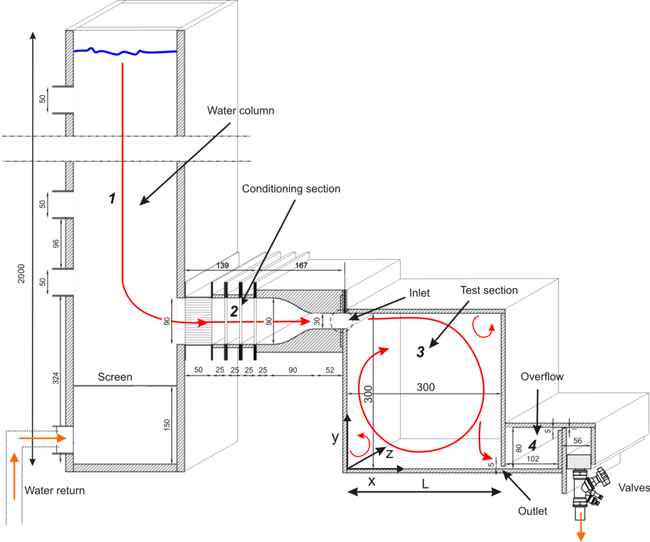Figure 2: Reduced-scale experimental setup: (1) water column; (2) flow conditioning section in front of the inlet, (3) test section, (4) overflow, and valves that are placed in a block after the overflow. Dimensions in mm. Figure modified from van Hooff et al. (2013).

### PIV setup and data files

Measurements were performed in the vertical center plane of the reduced-scale enclosure presented in the previous sections using PIV. More detailed information on the experimental setup can be found in van Hooff et al. (2012a, 2012b).

Measurements were performed using a 2D PIV system, measuring the horizontal (x-direction) and vertical (y-direction) velocity components (u,v). The PIV system consisted of a Nd:Yag (532 nm) double-cavity laser (2 x 200 mJ, repetition rate < 10 Hz) used to illuminate the field of view, and a CCD (Charge Coupled Device) camera (1376 x 1040 pixel resolution, 10 frames/s) for image acquisition. The laser was positioned above the cubic test section to create a laser sheet in the vertical center plane of the cube (z/L = 0.5); the camera was positioned perpendicular to the illuminated plane. Hollow glass micro spheres (3M; type K1) with diameters in the range of 30 – 115 μm were used to provide seeding. Two sets of PIV measurements were performed, both in the vertical center plane (z/L = 0.5). The first set focused on the entire vertical cross-section of the enclosure (0.3 x 0.3 m2 (= ROI1)) (Fig. 3), while a second set focused on a smaller area of 0.18 x 0.12 m2 (W x H) to obtain a higher measurement resolution near the inlet (= ROI2). The measuring frequency was 2 Hz and each measurement set consisted of 360 uncorrelated samples resulting in an averaging time of 180 seconds. Systematic errors were present for each sample and consisted of a range of errors that are associated with the PIV measurement technique and methodology (e.g. Prasad (2000)) and were minimized using the best practice guidelines of Keane and Adrian (1990) and Prasad (2000). The repeatability (or random) error is a statistical error, of which the uncertainty was assessed using the central limit theorem (e.g. Coleman and Steel 1999). The uncertainty of the measurement results was found to be around 2-4% in the largest part of the test section, whereas it was slightly higher in the shear layer and boundary layer areas due to the locally higher turbulence levels (van Hooff et al. (2012a)).

The experimental data files can be found here:

The files for ROI1 contain measured time-averaged x-velocities (U; m/s) along three vertical lines in the vertical center plane of the cube (z/L = 0.5), namely at x/L = 0.2, x/L = 0.5 and x/L = 0.8, while the files for ROI2 contain both time-averaged x-velocities (U; m/s) and turbulent kinetic energy values (k; m2/s2 - determined from the measured u- and v- fluctuations as explained in the Evaluation section) along vertical lines at x/L = 0.2 and x/L = 0.5. The first column contains the horizontal coordinate (x/L), the second column the vertical coordinate (y/L). All values are taken in the vertical center plane (z/L = 0.5).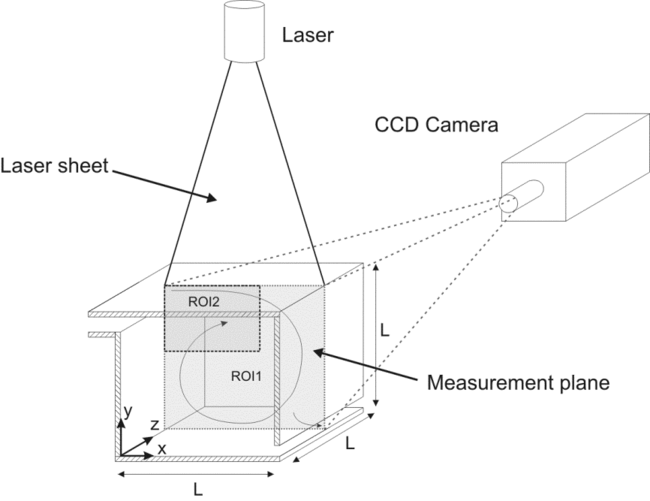Figure 3: PIV measurement setup; the laser head is positioned above the test section using a translation stage. ROI1 indicates the region of interest (0.3 x 0.3 m2) for the first measurement set, ROI2 indicates the smaller region of interest of 0.18 x 0.12 m2 (W x H) for the second set. Figure from van Hooff et al. (2012a).

### Results: transitional flow

It was assured that transitional flow was present by performing flow visualizations using fluorescent dye injected in the center of the inlet opening. Figure 4 shows an example of such visualization for slot Re numbers of Re ≈ 1,000 and Re ≈ 2,500, in which it is shown that the jet was laminar first and became transitional downstream, when shear layer instabilities started and vortical structures started to develop in the shear layer. The onset to transitional flow occurred further upstream with increasing Reynolds number. Figure 4 also shows that jet detachment from the top surface occurs further downstream with increasing Re, resulting in a slightly different overall flow pattern.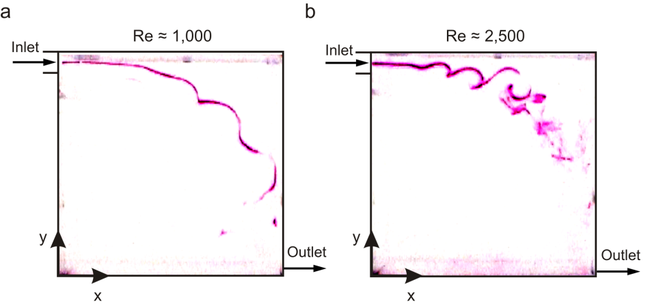Figure 4: Instantaneous images of the flow pattern in vertical centerplane of the enclosure. (a) Re ≈ 1,000. (b) Re ≈ 2,500. Figure modified from van Hooff et al. (2012a).

In addition, measured vertical profiles of U/UM were compared with the theoretical profiles of a laminar (Glauert 1956) and turbulent (Verhoff 1963) unconfined wall jet and the comparison is depicted in Figure 5. The comparison was made at five distances (x/L = 0.1, 0.2, 0.3, 0.4, 0.5). The non-dimensional y-coordinate is y/y½, with y/y½ = 0 at the top surface. Figure 5a shows that the velocity profiles are in relatively good agreement with the theoretical profile for a laminar wall jet for Re ≈ 1,000, although the measured profiles have an almost top-hat profile instead of a parabolic profile close to the inlet (x/L = 0.1 and x/L = 0.2), which are locations where the wall jet is not yet fully developed. At x = 0.5 the wall jet has developed into a jet with a parabolic profile, however, the maximum velocity is now located at a larger distance from the top surface compared to the profile of Glauert (1956). Figure 5b shows the comparison for Re ≈ 2,500; the measured profiles in the outer region resemble the theoretical profile for a laminar wall jet to a large extent, whereas those in the inner region are moving even further upwards to the top surface, and consequently also to the theoretical profile of a turbulent wall jet. Again, the velocity profiles close to the inlet are more or less top-hat shaped, indicating that the wall jet is not yet fully developed at these locations.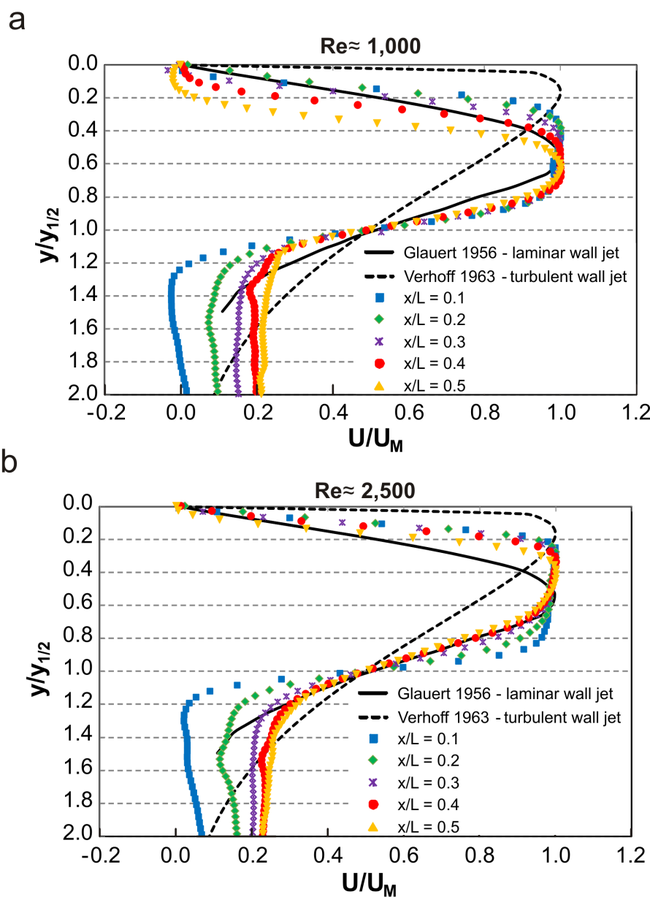Figure 5: Comparison between measured wall jet profiles and the theoretical profiles for a laminar (Glauert 1956) and a turbulent (Verhoff 1963) wall jet for: (a) Re ≈ 1,000; (b) Re ≈ 2,500. Figure modified from van Hooff et al. (2012a).

## CFD methods

Note that additional information on the CFD simulations can be obtained from van Hooff et al. (2013).

### Computational geometry and grid

The computational geometry replicated part of the experimental setup that was described in the previous sections. The contraction was included in the geometrical model (Fig. 6a), but not the honeycombs and the screens of the conditioning section. In addition, the outlet was extended in the x-direction to enhance stability and convergence of the simulations. A 3D model was used since preliminary tests indicated a clear difference in velocity profiles along three vertical lines in the vertical center plane when compared to 2D simulation results. The computational grid was created using the surface-grid extrusion technique presented in van Hooff and Blocken (2010). A grid-sensitivity analysis was conducted based on a linear grid refinement factor of √2 in each direction, resulting in three different fully structured grids with cell counts that ranged from 1,254,400 (Fig. 6b) to 10,022,400. The grid-sensitivity analysis was performed for Re ≈ 1,000 and with the SST k-ω model by Menter (1994). A higher grid resolution was applied in the boundary layer and shear layer. The number of cells over the inlet height was 40, 56 and 80 for the coarse, middle and fine grid, respectively. An overview of the grid size and the corresponding dimensionless wall distances y* at the top surface (y/L = 1) in the center plane is provided in Table 1. The y* values were used in this study instead of y+ values due to the problems in definition of y+ when flow is re-attaching or impinging; in these particular flows y+ will go to zero since ${\tau _{w}}$goes to zero. This problem is avoided when using y*, since y* is based on turbulent kinetic energy:

${y^{*}={\frac {\rho C_{\mu }^{1/4}k_{P}^{1/2}y_{P}}{\mu }}\qquad (3)}$with ${\rho }$the density, ${C_{\mu }}$the model constant, ${k_{P}}$the turbulent kinetic energy at the near-wall node P, ${y_{P}}$the distance from point P to the wall, and ${\mu }$the dynamic viscosity of the fluid. In several commercial CFD codes, among which ANSYS Fluent, wall treatment is based on y* rather than on y+ (e.g. Casey and Wintergerste 2000). Note that in equilibrium turbulent boundary layer flows y* = y+. The low values for y* as reported in Table 1 enabled the use of low-Reynolds number modeling (LRNM), i.e. solving the flow all the way down to the wall including the viscous sublayer. This is especially important in situations in which low-Reynolds-number flow, pressure gradients and boundary layer separation are present, as is the case in this UFR case.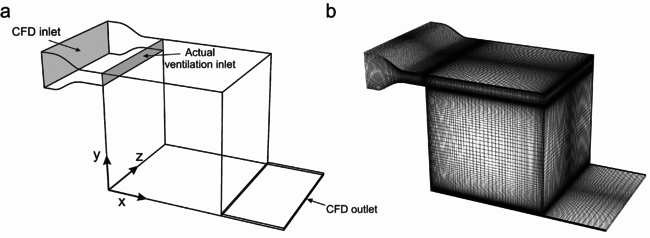Figure 6: (a) Computational model of the water cube. (b) Computational grid (coarse grid: 1.25 million cells). Figure from van Hooff et al. (2013).

The grid-sensitivity analysis showed that the results obtained with the coarse grid resulted in significant differences from the results obtained with the middle grid (3,437,056 cells), while the results of the fine grip were nearly identical to the results of the middle grid. More information can be found in van Hooff et al. (2013).

It must be noted that the simulations for the grid-sensitivity analysis showed oscillatory convergence, comparable to that reported by Ramponi and Blocken (2012) and van Hooff et al. (2012c). As a result, relatively small fluctuations of the velocity were observed in the wall jet region of about 2% to 5%. An average solution was obtained by averaging the results over a sufficiently large number of iterations. Overall, the middle grid (3,437,056 cells) provided fairly grid-independent results and was therefore used in the other simulations reported in this UFR case.

Table 1: Grids used for the grid-sensitivity analysis (Table from van Hooff et al. (2013)).
Grid Number of cells [-] y* top surface; min [-] y*top surface; max [-]
Coarse 1,254,400 0.045 0.45
Middle 3,437,056 0.026 0.40
Fine 10,022,400 0.007 0.28

### Boundary conditions

The wall surfaces were modeled as smooth no-slip walls. The values of ε and ω at the wall depend on the turbulence model and near-wall treatment chosen; more information can be found in the publications on turbulence models and near-wall treatment mentioned in the section on solver settings. A uniform velocity was imposed at the CFD inlet (see Fig. 6a), i.e. upstream of the contraction, based on the Reynolds number at the actual ventilation inlet during the experiments and the ratio between the height of the CFD inlet (h/L = 0.3) and the actual ventilation inlet (h/L = 0.1): Uinlet;CFD = 0.01 m/s for Re ≈ 1,000 and Uinlet;CFD = 0.025 m/s for Re ≈ 2,500. The turbulence parameters were specified based on the hydraulic diameter and the turbulence intensity. The hydraulic diameter Dh was calculated using Dh = (4WH)/(2(W+H)), with H the height and W the width of the CFD inlet. The turbulence intensity (uRMS/UM) in the wall jet region at x/L = 0.2 was around 3-4% for each value of Re (van Hooff et al. (2012a)). A constant turbulence intensity of 6% was imposed at the CFD inlet for Re ≈ 1,000 and 18% for Re ≈ 2,500. Due to the contraction the resulting turbulent kinetic energy levels downstream of the inlet showed a fair to good overall agreement with the measured values in the potential core region of the wall jet (see Evaluation). The values of k, ε and ω at the inlet were calculated based on the hydraulic diameter (Dh, with Dh = L, and l = 0.07L) and the imposed turbulence intensity:

${{\frac {3}{2}}\left(UI_{U}\right)^{2}\qquad (4)}$${\varepsilon =C_{\mu }^{\frac {3}{4}}{\frac {k^{\frac {3}{2}}}{l}}\qquad (5)}$${\omega ={\frac {k^{\frac {1}{2}}}{C_{\mu }^{\frac {1}{4}}l}}\qquad (6)}$Finally, zero static gauge pressure was imposed at the outlet.

### Solver settings

3D isothermal steady RANS simulations were performed with the commercial CFD code Fluent 6.3.26 (Fluent 2006). In this case, three RANS turbulence models were tested:

1. Low-Reynolds k-ω Chang et al. (1995)
2. SST k-ω Menter (1994)
3. Low-Re stress-omega Reynolds Stress Model Wilcox (1998)

The model constants were taken equal to the default model constants implemented in Fluent, which are not mentioned here for the sake of brevity (see Fluent User Guide 2006). All models are low-Reynolds number models that can be used until the wall boundary.

The Low-Reynolds number k-ω model by Chang et al. (1995) is developed for flows subjected to a sudden expansion (recirculating flows), which is the case in this UFR, and is therefore expected to be the most suitable low-Reynolds number version of the k-ω model for this flow problem. In addition, the low-Reynolds number k-ω turbulence model by Chang et al. (1995) solves all equations until the wall and applies damping functions in the equation for μt and in the transport equation for ε, which can lead to a more accurate prediction of the near-wall flow than with high-Reynolds number versions of the k-ω model, such as the RNG k-ω model by Yakhot et al. (1992).

Note that most RANS turbulence models were developed for fully developed turbulent flow, however, the measurements were conducted for transitional slot Reynolds numbers.

Pressure-velocity coupling was performed by the SIMPLEC algorithm, pressure interpolation was second order and second-order upwind discretization schemes were used for both the convection terms and the viscous terms of the governing equations. Convergence was monitored carefully. For the majority of the simulations oscillatory convergence was present. An average solution was obtained by averaging over an interval of 4,000 or 20,000 iterations, depending on the Re number and the turbulence model. Note that oscillatory convergence is more frequent in low-velocity indoor airflows than in strongly momentum-driven flows, however it is not always reported in publications of indoor airflow studies.

Contributed by: T. van Hooff(*), B. Blocken(*), G.J.F. van Heijst(**) — (*)Dept. of Civil Engineering, KU Leuven, Belgium and Dept. of the Built Environment, Eindhoven University of Technology, the Netherlands.
(**)Dept. of Applied Physics, Eindhoven University of Technology, the Netherlands